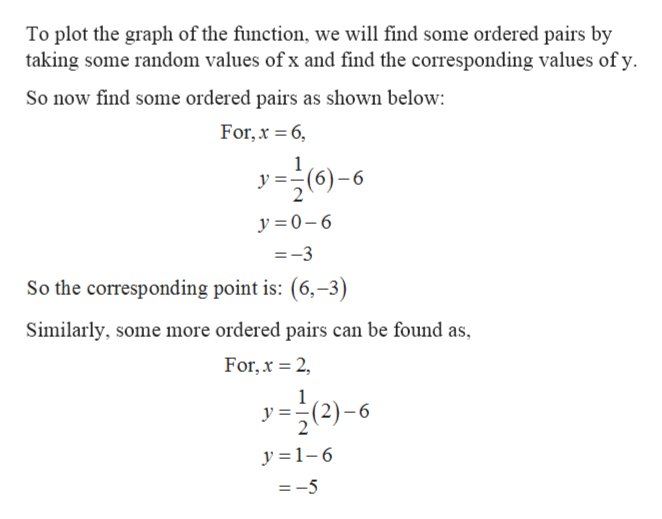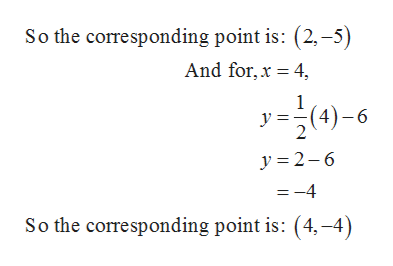Question
1 views

Graph by using the y-

check_circle

Step 1

To Graph of the given function.

Step 2

Calculation: -help_outlineImage TranscriptioncloseTo plot the graph of the function, we will find some ordered pairs by taking some random values of x and find the corresponding values of y. So now find some ordered pairs as shown below: For, x = 6, y ==(6)–6 y = 0-6 =-3 So the corresponding point is: (6,–3) Similarly, some more ordered pairs can be found as, For, x = 2, y=2)-6 y =1-6 = -5 fullscreen
Step 3help_outlineImage TranscriptioncloseSo the corresponding point is: (2, –5) And for, x = 4, y=;(4)-6 y = 2-6 = -4 So the corresponding point is: (4, –4) fullscreen

### Want to see the full answer?

See Solution

#### Want to see this answer and more?

Solutions are written by subject experts who are available 24/7. Questions are typically answered within 1 hour.*

See Solution
*Response times may vary by subject and question.
Tagged in
MathAlgebra

### Other